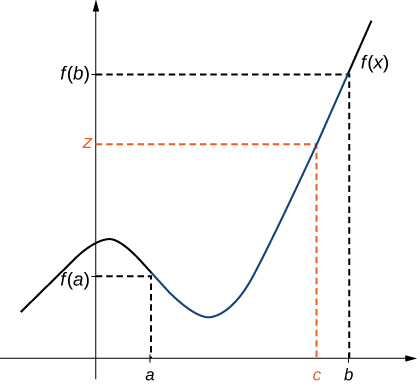# 2.4 Continuity  (Page 5/16)

 Page 5 / 16

## The intermediate value theorem

Let f be continuous over a closed, bounded interval $\left[a,b\right].$ If z is any real number between $f\left(a\right)$ and $f\left(b\right),$ then there is a number c in $\left[a,b\right]$ satisfying $f\left(c\right)=z$ in [link] .There is a number c ∈ [ a , b ] that satisfies f ( c ) = z .

## Application of the intermediate value theorem

Show that $f\left(x\right)=x-\text{cos}\phantom{\rule{0.1em}{0ex}}x$ has at least one zero.

Since $f\left(x\right)=x-\text{cos}\phantom{\rule{0.1em}{0ex}}x$ is continuous over $\left(\text{−}\infty ,\text{+}\infty \right),$ it is continuous over any closed interval of the form $\left[a,b\right].$ If you can find an interval $\left[a,b\right]$ such that $f\left(a\right)$ and $f\left(b\right)$ have opposite signs, you can use the Intermediate Value Theorem to conclude there must be a real number c in $\left(a,b\right)$ that satisfies $f\left(c\right)=0.$ Note that

$f\left(0\right)=0-\text{cos}\phantom{\rule{0.1em}{0ex}}\left(0\right)=-1<0$

and

$f\left(\frac{\pi }{2}\right)=\frac{\pi }{2}-\text{cos}\frac{\pi }{2}=\frac{\pi }{2}>0.$

Using the Intermediate Value Theorem, we can see that there must be a real number c in $\left[0,\pi \text{/}2\right]$ that satisfies $f\left(c\right)=0.$ Therefore, $f\left(x\right)=x-\text{cos}\phantom{\rule{0.1em}{0ex}}x$ has at least one zero.

## When can you apply the intermediate value theorem?

If $f\left(x\right)$ is continuous over $\left[0,2\right],f\left(0\right)>0$ and $f\left(2\right)>0,$ can we use the Intermediate Value Theorem to conclude that $f\left(x\right)$ has no zeros in the interval $\left[0,2\text{]?}$ Explain.

No. The Intermediate Value Theorem only allows us to conclude that we can find a value between $f\left(0\right)$ and $f\left(2\right);$ it doesn’t allow us to conclude that we can’t find other values. To see this more clearly, consider the function $f\left(x\right)={\left(x-1\right)}^{2}.$ It satisfies $f\left(0\right)=1>0,f\left(2\right)=1>0,$ and $f\left(1\right)=0.$

## When can you apply the intermediate value theorem?

For $f\left(x\right)=1\text{/}x,f\left(-1\right)=-1<0$ and $f\left(1\right)=1>0.$ Can we conclude that $f\left(x\right)$ has a zero in the interval $\left[-1,1\right]?$

No. The function is not continuous over $\left[-1,1\right].$ The Intermediate Value Theorem does not apply here.

Show that $f\left(x\right)={x}^{3}-{x}^{2}-3x+1$ has a zero over the interval $\left[0,1\right].$

$f\left(0\right)=1>0,f\left(1\right)=-2<0;f\left(x\right)$ is continuous over $\left[0,1\right].$ It must have a zero on this interval.

## Key concepts

• For a function to be continuous at a point, it must be defined at that point, its limit must exist at the point, and the value of the function at that point must equal the value of the limit at that point.
• Discontinuities may be classified as removable, jump, or infinite.
• A function is continuous over an open interval if it is continuous at every point in the interval. It is continuous over a closed interval if it is continuous at every point in its interior and is continuous at its endpoints.
• The composite function theorem states: If $f\left(x\right)$ is continuous at L and $\underset{x\to a}{\text{lim}}g\left(x\right)=L,$ then $\underset{x\to a}{\text{lim}}f\left(g\left(x\right)\right)=f\left(\underset{x\to a}{\text{lim}}g\left(x\right)\right)=f\left(L\right).$
• The Intermediate Value Theorem guarantees that if a function is continuous over a closed interval, then the function takes on every value between the values at its endpoints.

For the following exercises, determine the point(s), if any, at which each function is discontinuous. Classify any discontinuity as jump, removable, infinite, or other.

$f\left(x\right)=\frac{1}{\sqrt{x}}$

The function is defined for all x in the interval $\left(0,\infty \right).$

$f\left(x\right)=\frac{2}{{x}^{2}+1}$

$f\left(x\right)=\frac{x}{{x}^{2}-x}$

Removable discontinuity at $x=0;$ infinite discontinuity at $x=1$

$g\left(t\right)={t}^{-1}+1$

$f\left(x\right)=\frac{5}{{e}^{x}-2}$

Infinite discontinuity at $x=\text{ln}\phantom{\rule{0.1em}{0ex}}2$

$f\left(x\right)=\frac{|x-2|}{x-2}$

$H\left(x\right)=\text{tan}\phantom{\rule{0.1em}{0ex}}2x$

Infinite discontinuities at $x=\frac{\left(2k+1\right)\pi }{4},$ for $k=0,±1,±2,±3\text{,…}$

$f\left(t\right)=\frac{t+3}{{t}^{2}+5t+6}$

For the following exercises, decide if the function continuous at the given point. If it is discontinuous, what type of discontinuity is it?

any genius online ? I need help!!
Pina
need to learn polynomial
Zakariya
i will teach...
nandu
I'm waiting
Zakariya
evaluate the following computation (x³-8/x-2)
teach me how to solve the first law of calculus.
what is differentiation
f(x) = x-2 g(x) = 3x + 5 fog(x)? f(x)/g(x)
fog(x)= f(g(x)) = x-2 = 3x+5-2 = 3x+3 f(x)/g(x)= x-2/3x+5
diron
pweding paturo nsa calculus?
jimmy
how to use fundamental theorem to solve exponential
find the bounded area of the parabola y^2=4x and y=16x
what is absolute value means?
Chicken nuggets
Hugh
🐔
MM
🐔🦃 nuggets
MM
(mathematics) For a complex number a+bi, the principal square root of the sum of the squares of its real and imaginary parts, √a2+b2 . Denoted by | |. The absolute value |x| of a real number x is √x2 , which is equal to x if x is non-negative, and −x if x is negative.
Ismael
find integration of loge x
find the volume of a solid about the y-axis, x=0, x=1, y=0, y=7+x^3
how does this work
Can calculus give the answers as same as other methods give in basic classes while solving the numericals?
log tan (x/4+x/2)
Rohan
Rohan
y=(x^2 + 3x).(eipix)
Claudia
Ismael
A Function F(X)=Sinx+cosx is odd or even?
neither
David
Neither
Lovuyiso
f(x)=1/1+x^2 |=[-3,1]
apa itu?
fauzi
determine the area of the region enclosed by x²+y=1,2x-y+4=0
Hi
MP
Hi too
Vic
hello please anyone with calculus PDF should share
Which kind of pdf do you want bro?
Aftab
hi
Abdul
can I get calculus in pdf
Abdul
explain for me
Usman
okay I have such documents
Fitzgerald
Hamza

#### Get Jobilize Job Search Mobile App in your pocket Now!By Mahee BooBy Brooke DelaneyBy OpenStaxBy Brianna BeckBy OpenStaxBy Vanessa SoledadBy OpenStaxBy Jonathan LongBy LaToya TrowersBy Anh Dao